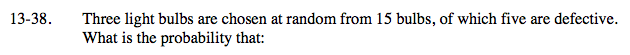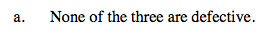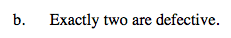### Home > A2C > Chapter 13 > Lesson 13.1.2 > Problem13-38

13-38.
1. Three light bulbs are chosen at random from 15 bulbs, of which five are defective. What is the probability that: Homework Help ✎

1. None of the three are defective.

2. Exactly two are defective.$\frac{_{10}C_3 \cdot _5C_0}{_{15}C_3}=\frac{24}{91}$Exactly two are defective means that one is not defective.

Use the method from part (a).